# Calculation of Junction Temperature, TJ

## Thermal Resistance

The following figure shows a conceptual diagram of thermal resistance, RθJC. The specified position of temperature depends on the package and product.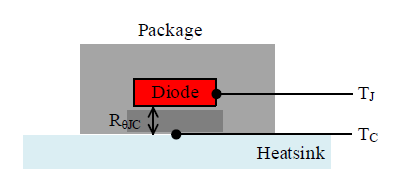Where:
TJ is junction temperature,
TC is case temperature, and
RθJC is thermal resistance between junction and case.

## Calculation from Case Temperature

Junction temperature, TJ is estimated from the measured values of loss and case temperature, TC during the power MOSFET/IGBT operation and the thermal resistance, RθJC described in the electrical characteristics of the data sheet. TJ is calculated by the following equations.

 TJ = P × RθJC + TC

Where:
P is the loss of the power MOSFET/IGBT (W),
RθJC is thermal resistance between junction and case (°C/W), and
TC is case temperature (°C).

As an example, when P = 0.6 W, RθJC = 20 °C/W, and TC = 80 °C, TJ is calculated by the following equation.

 TJ = 0.6 × 20 + 80 = 92 (°C)

## Calculation from Transient Thermal Resistance Characteristics

TJ when power is applied instantaneously is estimated from the transient thermal resistance data. TJ is calculated by the following equation.

 TJ = P × rθJC + TC

Where:
P is the loss of the power MOSFET/IGBT (W),
rθJC is transient thermal resistance between junction and case temperature (°C/W), and
TC is case temperature (°C).

The following figure shows the transient thermal resistance characteristics example. For example, when a single square pulse of 100 ms is applied, rθJC is 2 °C/W.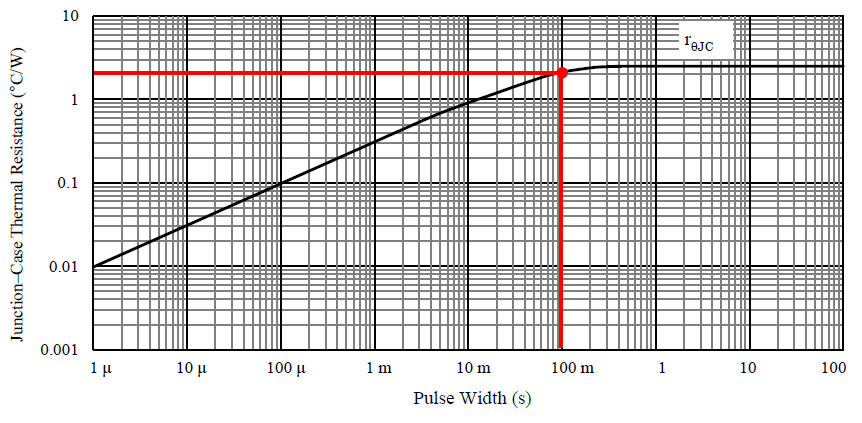For example, when P = 0.6 W, rθJC = 2 °C/W, and TC = 100 °C, TJ is calculated by the following equation.

 TJ = P × 0.6 × 2 + 100 = 101.2 (°C)

## Calculation Using Superposition Theorem

This section shows the calculation of the junction temperature, TJ, when a regular square wave power loss occurs in the power MOSFET/IGBT and the junction temperature, TJ, when an irregular square wave power loss occurs in the power MOSFET/IGBT. For such power loss waveforms, it is easy and effective to read the thermal resistance in each period from the transient thermal resistance characteristic graph in the data sheet and use the superposition theorem.

### Continuous Pulse

(A) shows the calculation of the junction temperature, TJ, when a regular square wave power loss occurs in the power MOSFET/IGBT. To easily calculate TJ, assume that power losses for two cycles occur in the average power loss over the entire period.
As shown in (B) and (C), TJ is calculated by approximating the power loss and using the superposition theorem.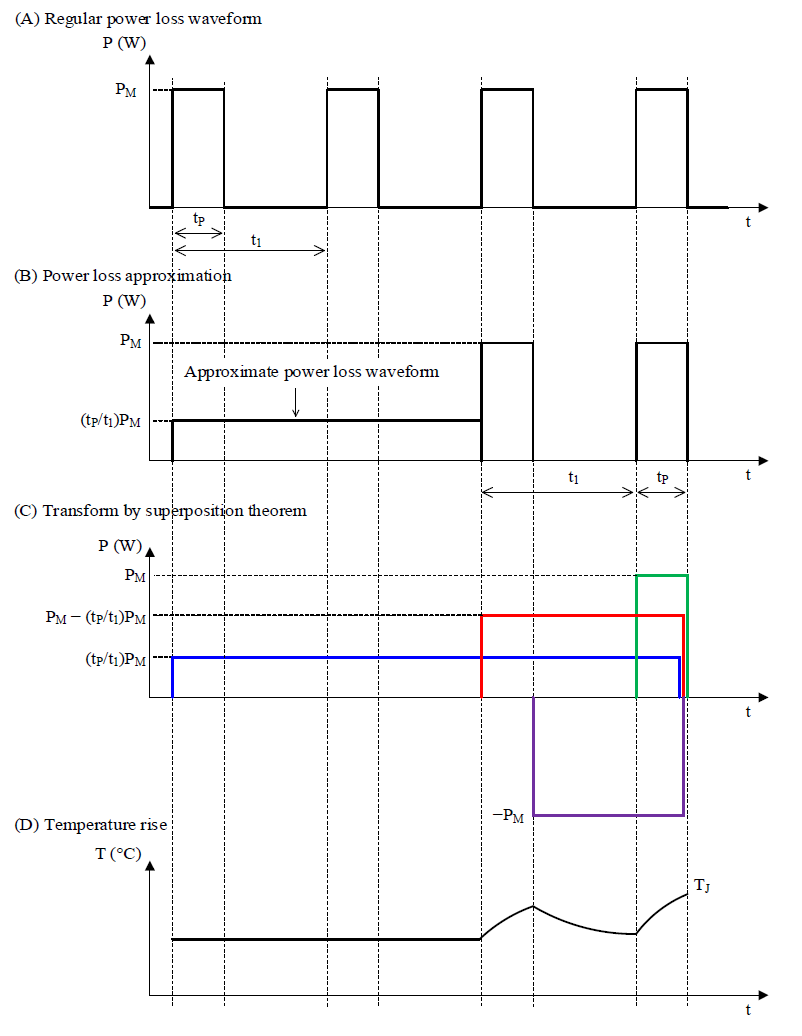Next, the junction temperature is calculated for each block in (C).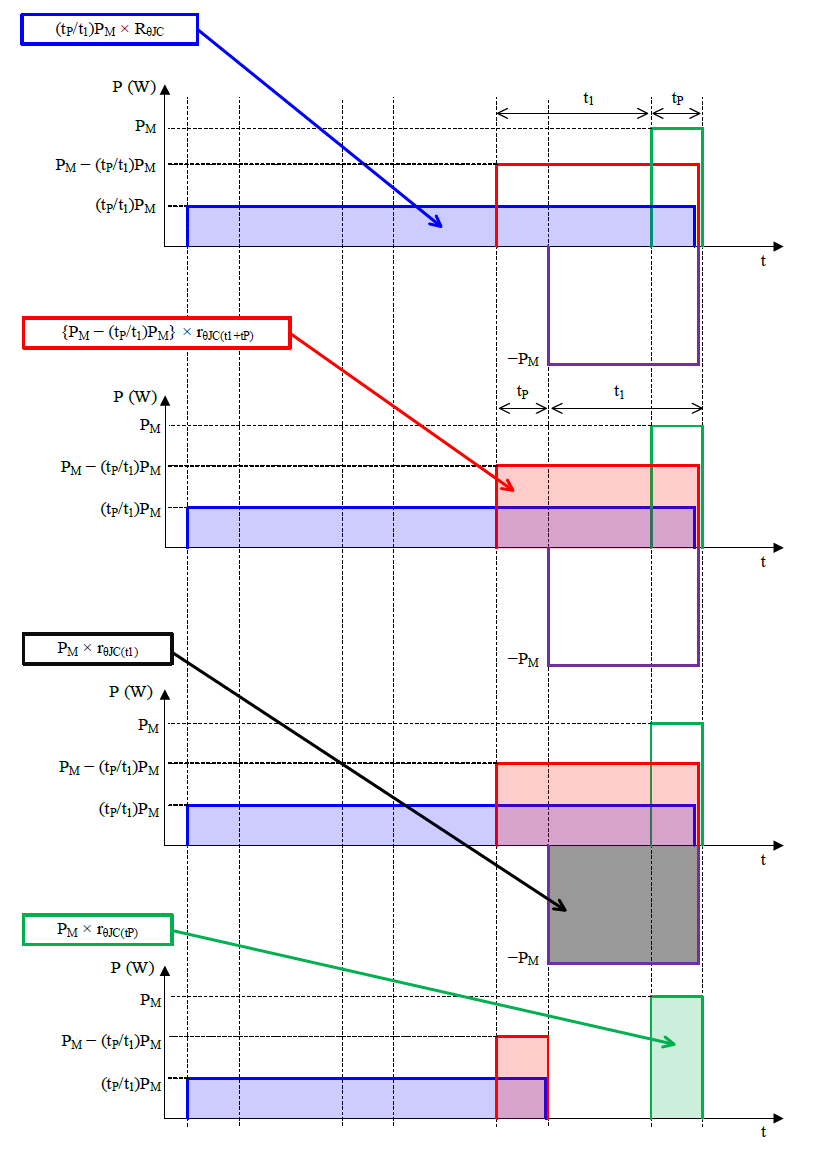Thus, TJ when using the superposition theorem is calculated by the following equation.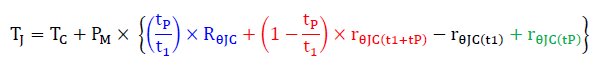Where:
TC is case temperature (°C),
PM is average power loss (W),
t1 is period (s),
tP is pulse width (s),
RθJC is the steady-state thermal resistance between junction and case temperature (°C/W),
rθJC(t1+tP) is the thermal resistance between junction and case temperature in the period, t1+tP (°C/W),
rθJC(t1) is the thermal resistance between junction and case temperature in the period, t1 (°C/W), and
rθJC(tP) is the thermal resistance between junction and case temperature in the period, tP (°C/W).

### Irregular Pulse

(A) shows the calculation of the junction temperature, TJ, when an irregular square wave power loss occurs in the power MOSFET/IGBT. As shown in (B), TJ for each block is calculated by using the superposition theorem.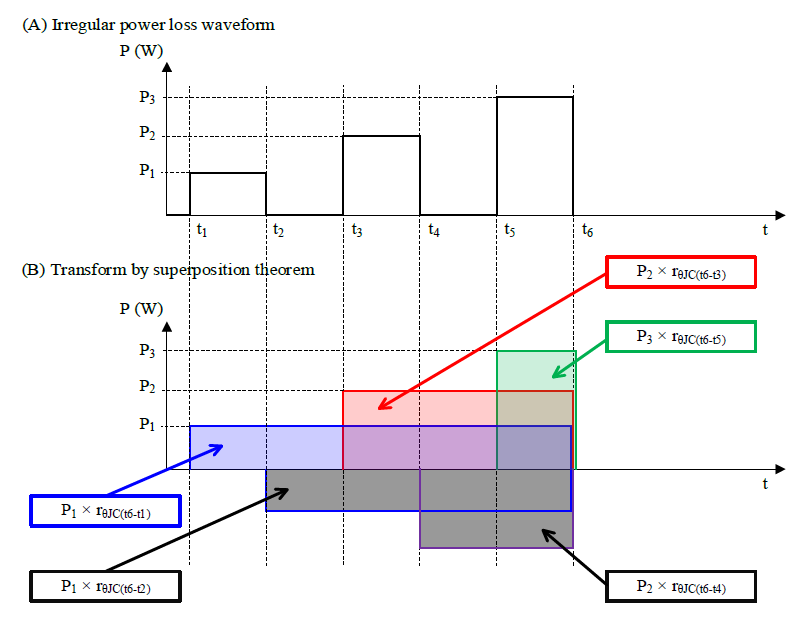Thus, TJ when using the superposition theorem is calculated by the following equation.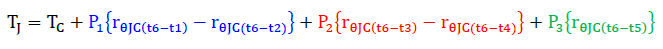Where:
TC is case temperature (°C),
P1 to P3 is power loss for each pulse (W),
rθJC(t6−t1) is the thermal resistance between junction and case temperature in the period, t6−t1 (°C/W),
rθJC(t6−t2) is the thermal resistance between junction and case temperature in the period, t6−t2 (°C/W),
rθJC(t6−t3) is the thermal resistance between junction and case temperature in the period, t6−t3 (°C/W),
rθJC(t6−t4) is the thermal resistance between junction and case temperature in the period, t6−t4 (°C/W), and
rθJC(t6−t5) is the thermal resistance between junction and case temperature in the period, t6−t5 (°C/W).